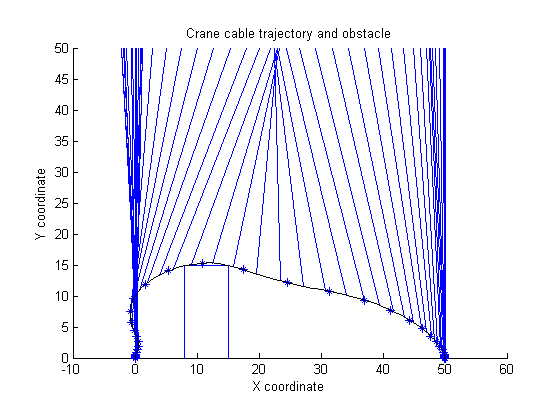55  Jumbo Crane Container Control

55.1  Problem description

Time-optimal control of a Jumbo Container Crane avoiding an obstacle.

Crane dynamics described in the book: Informatics in control automation and robotics II DOI 10.1007/978-1-4020-5626-0 , Springer, 2007, pp.79-84. T.J.J. van den Boom, J.B. Klaassens, R. Meiland Real-time optimal control for a non linear container crane using a neural network

Optimal control problem by: W.L. De Koning, G Fitie and L.G. Van Willigenburg

Programmers: Gerard Van Willigenburg (Wageningen University) Willem De Koning (retired from Delft University of Technology)

55.2  Problem setup

% Array with consecutive number of collocation points
narr = [7 10 40];

toms t t_f % Free final time

toms ho1

for i=1:length(narr)
p = tomPhase('p', t, 0, t_f, narr(i), [], 'cheb');
setPhase(p)

tomStates x1 x2 x3 x4 x5 x6
tomControls u1 u2

x = [x1; x2; x3; x4; x5; x6];

% Crane parameters
gr = 9.81;  He = 50;    Jh = 35.6;  Jt = 13.5;  ht = 50;
mc = 47000; mt = 33000; Nh = 26.14; Nt = 16.15; rh = 0.6; rt = 0.5;
xo_l = 8;    xo_r = 15;   hob = 15;

% Initial & terminal states
xi = [0;  0; 0; 0; 50; 0];
xf = [50; 0; 0; 0; 50; 0];

% Initial guess
if i==1;
x0 = {t_f==20.4; ho1==0; icollocate({x1 == 50*t/20; x2 == xi(2)
x3 == xi(3); x4 == xi(4); x5 == xi(5); x6 == xi(6)})
collocate({u1 == -2000; u2 == -5000})};
else
x0 = {t_f==tfopt;
icollocate({x1 == xopt1; x2 == xopt2
x3 == xopt3; x4 == xopt4; x5 == xopt5; x6 == xopt6})
collocate({u1 == uopt1; u2 == uopt2})};
end

% Box constraints
cbox = {15 <= t_f <= 30; -4200 <= collocate(u1) <= 4200
-11490 <= collocate(u2) <= 11490};

% Boundary constraints
cbnd = {initial(x == xi), final(x == xf)};

Gt  = Jt*Nt*Nt/(rt*rt); Gh = Jh*Nh*Nh/(rh*rh);
st  = sin(x3); ct = cos(x3);
Ft  = (Nt/rt)*u1; Fh = (Nh/rh)*u2;
d2x = (mc+Gh)*Ft-mc*Fh.*st+mc*gr*Gh*st.*ct+mc*Gh*x5.*x4.*x4.*st;
d2x = d2x./((mc+Gh)*(mt+Gt)+mc*Gh*(1-ct.*ct));

% xc is the container x position against time
xc = x1+x5*sin(x3);
% hc is the height of the container against time
hc = ht-x5*cos(x3);
% ho is the height of the obstacle at the container x position.
%ho = ifThenElse(xc<=xo_l,0,ifThenElse(xc>=xo_r,0,hob));
% do is the distance to the obstacle from the container.
do = max(max(xo_l-xc,xc-xo_r),hc-ho1);

% Path constraint - Distance to obstacle should always be >= 0
% and height should always be >= 0.
% Test 300 points, evenly spaced in time.
pth = {atPoints(linspace(0,t_f,300),{do>=0,hc>=0}),ho1>=hob};

% ODEs
ode = collocate({
dot(x1) == x2
dot(x2) == d2x
dot(x3) == x4
dot(x4) == (-2*x6.*x4-gr*st-d2x.*ct)./x5
dot(x5) == x6
dot(x6) == (Fh+mc*x5.*x4.*x4+mc*gr*ct-mc*d2x.*st)/(mc+Gh)
});

% Objective
objective = t_f;

55.3  Solve the problem

options = struct;
if i==1
% To improve convergece, we make the obstacle constraint softer,
% by including it in the ojbective rather than as a hard constraint
% in the first iteration.
% This is necessary because of the very nonlinear properties of this
% constraint.
pth = pth{1};
objective = objective - 2*ho1;
end
options.name = 'Crane with obstacle';
options.Prob.SOL.optPar(30) = 20000;
solution = ezsolve(objective, {cbox, cbnd, pth, ode}, x0, options);

tfopt = subs(t_f,solution);
xopt1 = subs(x1,solution);
xopt2 = subs(x2,solution);
xopt3 = subs(x3,solution);
xopt4 = subs(x4,solution);
xopt5 = subs(x5,solution);
xopt6 = subs(x6,solution);
uopt1 = subs(u1,solution);
uopt2 = subs(u2,solution);
Problem type appears to be: lpcon
Starting numeric solver
===== * * * =================================================================== * * *
TOMLAB - Tomlab Optimization Inc. Development license  999001. Valid to 2011-02-05
=====================================================================================
Problem: ---  1: Crane with obstacle            f_k     -44.290334943951777000
sum(|constr|)      0.000000001680375175
f(x_k) + sum(|constr|)    -44.290334942271400000
f(x_0)     20.399999999999999000

Solver: snopt.  EXIT=0.  INFORM=1.
SNOPT 7.2-5 NLP code
Optimality conditions satisfied

FuncEv    1 ConstrEv  387 ConJacEv  385 Iter   56 MinorIter 7258
CPU time: 5.812500 sec. Elapsed time: 5.890000 sec.
Problem type appears to be: lpcon
Starting numeric solver
===== * * * =================================================================== * * *
TOMLAB - Tomlab Optimization Inc. Development license  999001. Valid to 2011-02-05
=====================================================================================
Problem: ---  1: Crane with obstacle            f_k      21.780681675931579000
sum(|constr|)      0.000041663897094514
f(x_k) + sum(|constr|)     21.780723339828672000
f(x_0)     30.000000000000000000

Solver: snopt.  EXIT=0.  INFORM=1.
SNOPT 7.2-5 NLP code
Optimality conditions satisfied

FuncEv    1 ConstrEv  181 ConJacEv  180 Iter   62 MinorIter  768
CPU time: 2.750000 sec. Elapsed time: 2.969000 sec.
Problem type appears to be: lpcon
Starting numeric solver
===== * * * =================================================================== * * *
TOMLAB - Tomlab Optimization Inc. Development license  999001. Valid to 2011-02-05
=====================================================================================
Problem: ---  1: Crane with obstacle            f_k      19.487670710700066000
sum(|constr|)      0.000027250297174480
f(x_k) + sum(|constr|)     19.487697960997242000
f(x_0)     21.780681675931579000

Solver: snopt.  EXIT=0.  INFORM=1.
SNOPT 7.2-5 NLP code
Optimality conditions satisfied

FuncEv    1 ConstrEv  531 ConJacEv  529 Iter   74 MinorIter 1118
CPU time: 20.859375 sec. Elapsed time: 21.062000 sec.
end

% Get solution for 50 points, evenly distributed in time.
nt = 50;
topt = linspace(0,subs(t_f,solution),nt);
xopt = subs(atPoints(topt,x),solution);

% Plot
[nt,nx]=size(xopt);

clf
axis([-10 60 0 50]);
axis image;

% Draw Obstacle
line([xo_l xo_l xo_r xo_r],[0 hob hob 0]);
title('Crane cable trajectory and obstacle');

xtop=xopt(:,1); ytop=50*ones(size(topt));
xbottom=xopt(:,1)+xopt(:,5).*sin(xopt(:,3));
ybottom=50-xopt(:,5).*cos(xopt(:,3));

% Draw cable trajectory
tl=5; toptlen=length(topt);
for k=1:toptlen
line([xtop(k) xbottom(k)],[ytop(k) ybottom(k)]);
if tl/toptlen>0.01; pause(tl/toptlen); end
end
hold on; ezplot(xc,hc); hold off
xlabel('X coordinate'); ylabel('Y coordinate');